## A Discrete Time Markov Chain (DTMC) SIR Model in RImage Credit: Doug Buckley of http://hyperactive.to

There are many different techniques that be used to model physical, social, economic, and conceptual systems. The purpose of this post is to show how the Kermack-McKendrick (1927) formulation of the SIR Model for studying disease epidemics (where S stands for Susceptible, I stands for Infected, and R for Recovered) can be easily implemented in R as a discrete time Markov Chain using the markovchain package.

A Discrete Time Markov Chain (DTMC) is a model for a random process where one or more entities can change state between distinct timesteps. For example, in SIR, people can be labeled as Susceptible (haven’t gotten a disease yet, but aren’t immune), Infected (they’ve got the disease right now), or Recovered (they’ve had the disease, but no longer have it, and can’t get it because they have become immune). If they get the disease, they change states from Susceptible to Infected. If they get well, they change states from Infected to Recovered. It’s impossible to change states between Susceptible and Recovered, without first going through the Infected state. It’s totally possible to stay in the Susceptible state between successive checks on the population, because there’s not a 100% chance you’ll actually be infected between any two timesteps. You might have a particularly good immune system, or maybe you’ve been hanging out by yourself for several days programming.

Discrete time means you’re not continuously monitoring the state of the people in the system. It would get really overwhelming if you had to ask them every minute “Are you sick yet? Did you get better yet?” It makes more sense to monitor individuals’ states on a discrete basis rather than continuously, for example, like maybe once a day. (Ozgun & Barlas (2009) provide a more extensive illustration of the difference between discrete and continuous modeling, using a simple queuing system.)

To create a Markov Chain in R, all you need to know are the 1) transition probabilities, or the chance that an entity will move from one state to another between successive timesteps, 2) the initial state (that is, how many entities are in each of the states at time t=0), and 3) the markovchain package in R. Be sure to install markovchain before moving forward.

Imagine that there’s a 10% infection rate, and a 20% recovery rate. That implies that 90% of Susceptible people will remain in the Susceptible state, and 80% of those who are Infected will move to the Recovered Category, between successive timesteps. 100% of those Recovered will stay recovered. None of the people who are Recovered will become Susceptible.

Say that you start with a population of 100 people, and only 1 is infected. That means your “initial state” is that 99 are Susceptible, 1 is Infected, and 0 are Recovered. Here’s how you set up your Markov Chain:

```library(markovchain)
mcSIR <- new("markovchain", states=c("S","I","R"),
transitionMatrix=matrix(data=c(0.9,0.1,0,0,0.8,0.2,0,0,1),
byrow=TRUE, nrow=3), name="SIR")
initialState <- c(99,1,0)
```

At this point, you can ask R to see your transition matrix, which shows the probability of moving FROM each of the three states (that form the rows) TO each of the three states (that form the columns).

```> show(mcSIR)
SIR
A  3 - dimensional discrete Markov Chain with following states
S I R
The transition matrix   (by rows)  is defined as follows
S   I   R
S 0.9 0.1 0.0
I 0.0 0.8 0.2
R 0.0 0.0 1.0
```

You can also plot your transition probabilities:

`plot(mcSIR,package="diagram")`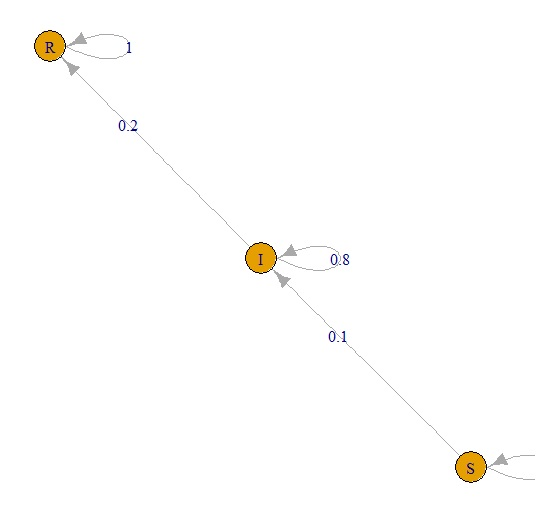But all we’ve done so far is to create our model. We haven’t yet done a simulation, which would show us how many people are in each of the three states as you move from one discrete timestep to many others. We can set up a data frame to contain labels for each timestep, and a count of how many people are in each state at each timestep. Then, we fill that data frame with the results after each timestep i, calculated by initialState*mcSIR^i:

```timesteps <- 100
sir.df <- data.frame( "timestep" = numeric(),
"S" = numeric(), "I" = numeric(),
"R" = numeric(), stringsAsFactors=FALSE)
for (i in 0:timesteps) {
newrow <- as.list(c(i,round(as.numeric(initialState * mcSIR ^ i),0)))
sir.df[nrow(sir.df) + 1, ] <- newrow
}```

Now that we have a data frame containing our SIR results (sir.df), we can display them to see what the values look like:

```> head(sir.df)
timestep  S  I  R
1        0 99  1  0
2        1 89 11  0
3        2 80 17  2
4        3 72 22  6
5        4 65 25 10
6        5 58 26 15
```

And then plot them to view our simulation results using this DTMC SIR Model:

```plot(sir.df\$timestep,sir.df\$S)
points(sir.df\$timestep,sir.df\$I, col="red")
points(sir.df\$timestep,sir.df\$R, col="green")```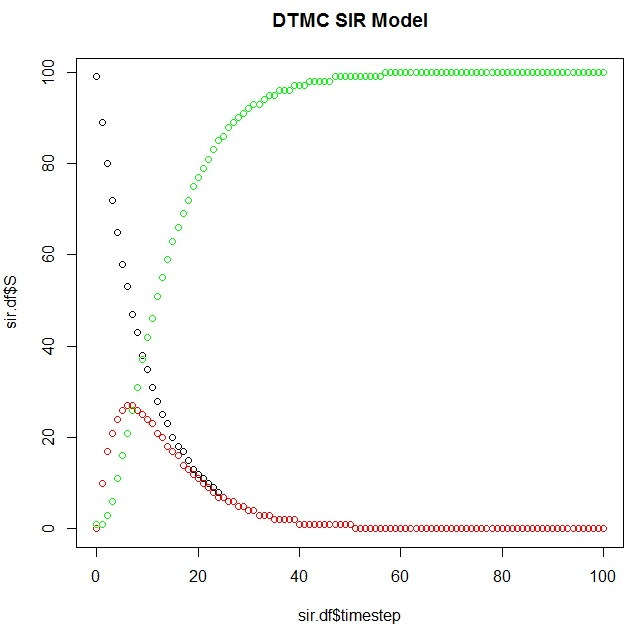It’s also possible to use the markovchain package to identify elements of your system as it evolves over time:

```> absorbingStates(mcSIR)
 "R"
> transientStates(mcSIR)
 "S" "I"
> steadyStates(mcSIR)
S I R
[1,] 0 0 1
```

And you can calculate the first timestep that your Markov Chain reaches its steady state (the “time to absorption”), which your plot should corroborate:

```> ab.state <- absorbingStates(mcSIR)
> occurs.at <- min(which(sir.df[,ab.state]==max(sir.df[,ab.state])))
> (sir.df[row,]\$timestep)+1
 58
```

You can use this code to change the various transition probabilities to see what the effects are on the outputs yourself (sensitivity analysis). Also, there are methods you can use to perform uncertainty analysis, e.g. putting confidence intervals around your transition probabilities. We won’t do either of these here, nor will we create a Shiny app to run this simulation, despite the significant temptation.

## Logistic Growth, S Curves, Bifurcations, and Lyapunov Exponents in R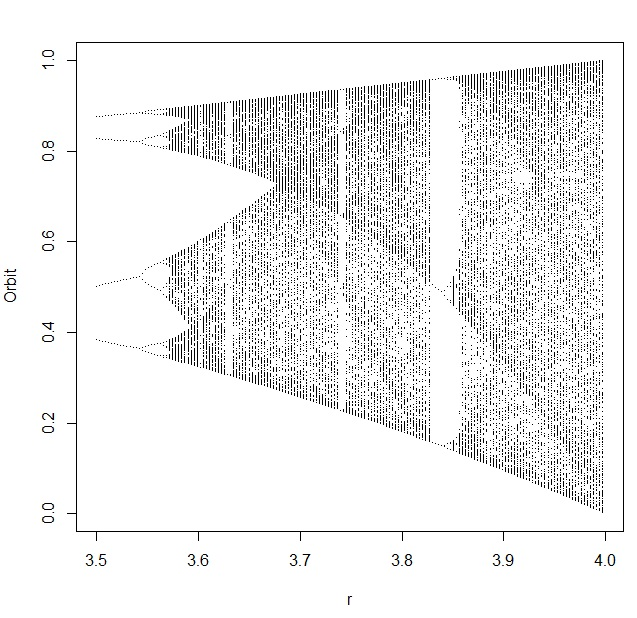If you’ve ever wondered how logistic population growth (the Verhulst model), S curves, the logistic map, bifurcation diagrams, sensitive dependence on initial conditions, “orbits”, deterministic chaos, and Lyapunov exponents are related to one another… this post explains it in just 10 steps, each with some code in R so you can explore it all yourself. I’ve included some code written by other people who have explored this problem (cited below) as portions of my own code.

It all starts with a hypothesized population… and a process where the size of the population changes over time. We want to understand how (and under what conditions) those changes occur, so we choose a model that characterizes population changes: the logistic growth model. It’s been used in biology, ecology, econometrics, marketing, and other areas.

1. The logistic growth model describes how the size of a population (N) changes over time (t), based on some maximum population growth rate (r). There is a limiting factor called the carrying capacity (K) which represents the total population that the environment could support, based on the amount of available resources. dN/dt is the rate of change of the population over time.2. You can simplify the logistic growth model by defining a new variable x to represent the portion of the population that’s alive, compared to the total population that the environment could support (and keep alive). So with x = N/K, you get a new differential equation in terms of x. Now we are looking at the rate of change of the population fraction over time. Once x = N/K = 1, the environment can’t support any more members in the population: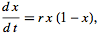3. You can solve this equation by integration! Then, you’ll have an expression that you can use to calculate x (which is still the population fraction) for any time t. This is called the sigmoid or (more commonly), the S Curve. To compute x at any time t, all we need to know is how big the population was when we started looking at it (x0) and the maximum growth rate r:4. The equation for the S Curve is deterministic and continuous. If we want to solve it numerically, we have to discretize it by chopping up that continuous axis that contains time into little tiny pieces of time. That’s what produces the difference equation that we recognize as the logistic map. It’s a map because it “maps” each value of the sequence onto the next value in the sequence. As long as you know one of those values for x (indicated by the subscript n), you’ll be able to figure out the next value of x (indicated by the subscript n+1). The value x[n] is the population fraction of the current generation, and the value x[n+1] is the population fraction for the next generation. This makes the logistic map a Markov chain. If you plot x[n] on the x axis and x[n+1] on the y axis, this expression will produce the familiar upside down parabola: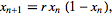5. The logistic map behaves differently depending upon the maximum growth rate (r) that describes your population. This parameter is also called fecundity and represents how rabbit-like your population is reproducing. The higher the r, the more productive, like rabbits (although I’m not sure precisely which r you’d choose if you were studying rabbits). Here is an R function that you can use to generate the last M iterations from a sequence of N total, developed and described at Mage’s Blog:

```logistic.map <- function(r, x, N, M) {
## from http://www.magesblog.com/2012/03/logistic-map-feigenbaum-diagram.html
## r: bifurcation parameter
## x: initial value, something greater than 0 and less than 1
## N: number of iterations (total)
## M: number of iteration points to be returned
z <- 1:N
z <- x
for(i in c(1:(N-1))){
z[i+1] <- r *z[i] * (1 - z[i])
}
## Return the last M iterations
z[c((N-M):N)]
}```

6. The logistic map has many interesting properties, but here are two in particular (the first in Step 6 and the second in Step 7). First, for several values you can choose for r, the chain converges to a single value (or fixed point) when n gets really big. For other values of r, the value of x will eventually bounce between two values instead of converging (a limit cycle of 2). For other values of r, the value of x will eventually bounce between four values instead of converging. Sometimes, x will bounce around a near limitless collection of values (a condition called deterministic chaos). The eventual values (or collection of eventual values, if they bounce between values) is called an orbit. For example, when the growth rate r is 2.6, the logistic map rapidly converges to an orbit of about 0.615:

`plot(logistic.map(2.6,.01,20,20), type="l")`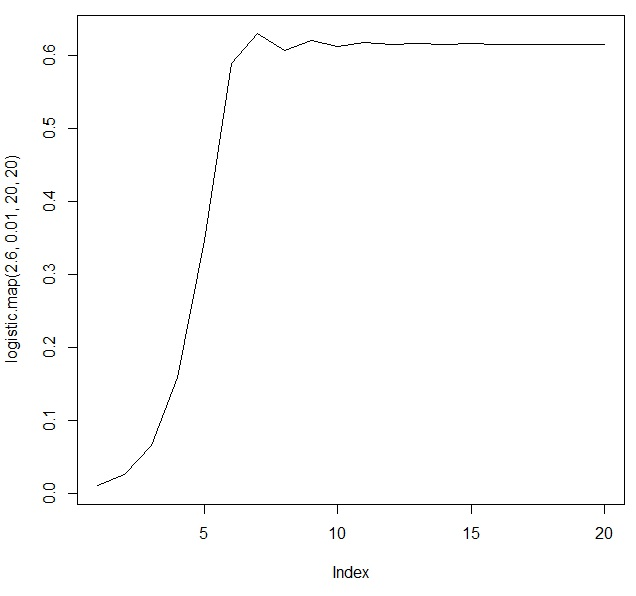7. Sometimes, it can be nice to take a look at how the values bounce around, and where they eventually converge (or not). To do this, we use cobweb diagrams (which are also sometimes called web diagrams). I used a function that I found at http://bayesianbiologist.com to plot the behavior of the orbits for r=2.6, r=3.2, and r=3.9:

```logistic.cobweb <- function(r) {
# code adapted from http://bayesianbiologist.com/tag/cobweb-plot/
x<-seq(0,1,length=100)
x_next <- lapply(2:N, function(i) r*x[i]*(1-x[i]))
plot(x[2:N],x_next,type="l",xlim=c(0,1), ylim=c(0,1), main=paste("r=",r),
xlab=expression(x[t]),ylab=expression(x[t+1]), col="red", lwd=2)
abline(0,1)

# start at your random spot on the x-axis and start with a vertical line:
start=runif(1,0,1)
vert=FALSE
lines(x=c(start,start),y=c(0,r*start*(1-start)) )
for(i in 1:(2*N)) {
if(vert) {
lines(x=c(start,start),y=c(start,r*start*(1-start)) )
vert=FALSE
} else {
lines(x=c(start, r*start*(1-start)), y=c(r*start*(1-start), r*start*(1-start)) )
vert=TRUE
start=r*start*(1-start)
}
}
}

par(mfrow=c(1,3))
logistic.cobweb(2.6)
logistic.cobweb(3.3)
logistic.cobweb(3.9)```8. (Remember to dev.off() before you continue.) Second, for some values of r, the logistic map shows sensitive dependence on initial conditions. For example, let’s see what happens for two different growth rates (r=3 and r=3.9) when we start one iteration with an x[n]  of 0.5 COLORED BLACK, and another one with an x[n] of 0.5001 COLORED RED. It’s a small, small difference that can lead to big, BIG variations in the orbits. In the r=3 case, the chain produced by the logistic map with x[n] of 0.5 (in black) is IDENTICAL to the chain produced by the logistic map with x[n] of 0.5001 (in red). That’s why you can’t see the black… the values are the same! But for the r=3.9 case, the chain produced by the logistic map with x[n] of 0.5 (in black) RAPIDLY DIVERGES from the chain produced by the logistic map with x[n] of 0.5001 (in red). They are very different, despite a very tiny difference in initial conditions! The logistic map for r=3.9 shows a very sensitive dependence on initial conditions

```par(mfrow=c(2,1))
first <- logistic.map(3,.5,120,100)
second <- logistic.map(3,.5001,120,100)
plot(1:length(first),first,type="l",main="r=3 is not sensitive to initial conditions")
lines(1:length(second),second,type="l",col="red")
first <- logistic.map(3.9,.5,120,100)
second <- logistic.map(3.9,.5001,120,100)
plot(1:length(first),first,type="l",main="but r=3.9 is EXTREMELY sensitive")
lines(1:length(second),second,type="l",col="red")

```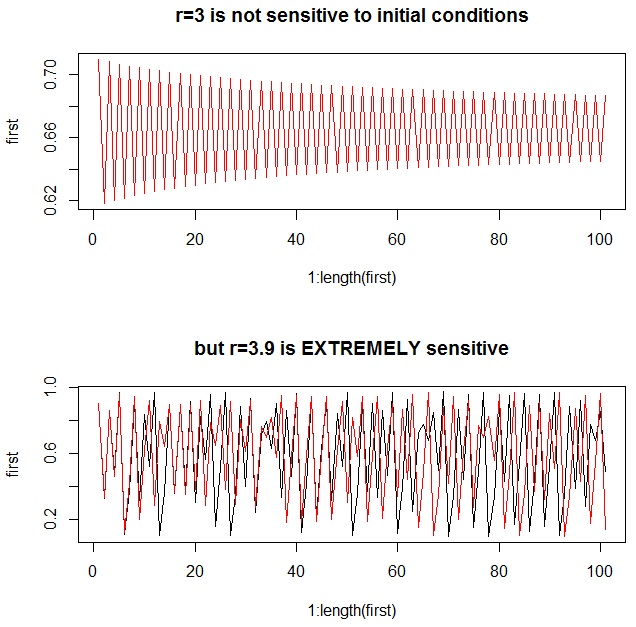9. For any chain, we can determine just how sensitive the logistic map is to initial conditions by looking at the Lyapunov exponent. Very simplistically, if the Lyapunov exponent is negative, the chain will converge to one or more fixed points for that value of r. If the Lyapunov exponent is positive, the chain will demonstrate deterministic chaos for that value of r. If the Lyapunov exponent is zero, there is a bifurcation: a 1-cycle is doubling to a 2-cycle, a 2-cycle is doubling to a 4-cycle, or so forth. The top chart shows an approximation of the Lyapunov exponent based on the first 500 iterations (ideally, you’d use an infinite number, but that would eat up too much computing time), and the bottom chart shows a bifurcation diagramYou’ll notice that the Lyapunov exponents are zero where a bifurcation occurs. To interpret the bifurcation diagram, just remember that each vertical slice through it represents the results of ONE COMPLETELY CONVERGED CHAIN from the logistic map. So it shows the results from many, many, many completely converged chains – and provides an excellent way for us to look at the behavior of MANY different types of populations in just one chart:

```n <- 400
XI <- lya <- 0
x <- seq(0,4,0.01)
for (i in 1:n) {
xi <- logistic.map(x[i],.01,500,500)
XI <- rbind(XI,xi)
}
for (i in 1:length(x)) {
lya[i] <- sum(log(abs(x[i]-(2*x[i]*XI[i,]))))/length(x)
}
plot(x,lya,ylim=c(-4,1),xlim=c(0,4),type="l",main="Lyapunov Exponents for Logistic Map")
abline(h=0, lwd=2, col="red")
# next 3 lines from http://www.magesblog.com/2012/03/logistic-map-feigenbaum-diagram.html:
my.r <- seq(0, 4, by=0.003)
Orbit <- sapply(my.r, logistic.map, x=0.1, N=1000, M=300)
r <- sort(rep(my.r, 301))

par(mfrow=c(2,1))
plot(x,lya,ylim=c(-5,1),xlim=c(0,4),type="l",main="Lyapunov Exponents for Logistic Map")
abline(h=0, col="red", lwd=2)
abline(v=3, col="blue", lwd=2)
plot(r, Orbit, pch=".", cex=0.5, main="Bifurcation Diagram for r=0 to r=4 Logistic Maps")
abline(v=3, col="blue", lwd=2)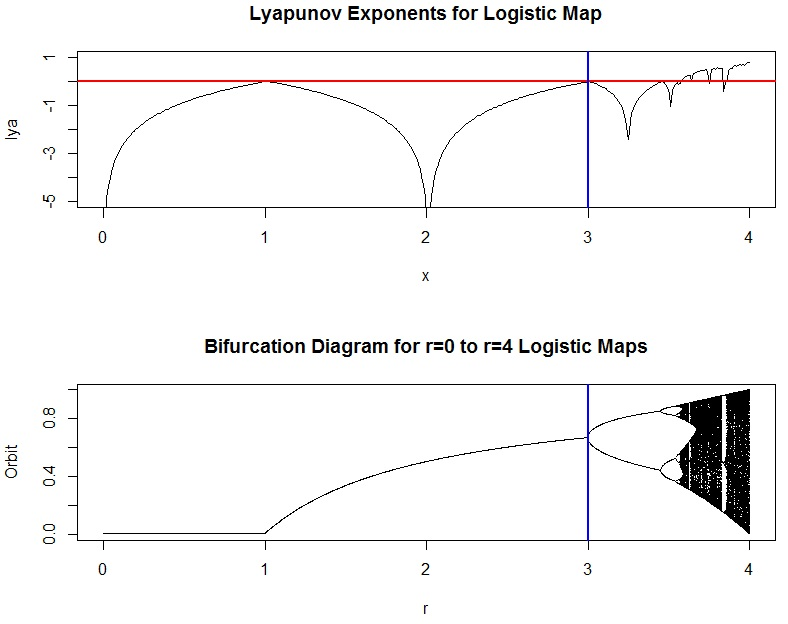```

10. Notice that in the bifurcation diagram, we can easily see that when r is between 0 and 1, the population converges to extinction. This makes sense, because the growth rate is smaller than what’s required to sustain the size of the population. You might like to zoom in, though, and see what the orbits look like for some smaller portions of the diagram. Here’s how you can do it (but be sure to refresh your graphics window with dev.off() before you try it). Try changing the plot character (pch) too, or maybe the size of the characters with cex=0.2 or cex=0.5 in the last line:

```# adapted from http://www.magesblog.com/2012/03/logistic-map-feigenbaum-diagram.html:
my.r <- seq(3.5, 4, by=0.003)
Orbit <- sapply(my.r, logistic.map, x=0.1, N=1000, M=300)
multiplier <- length(Orbit)/length(my.r)
r <- sort(rep(my.r, multiplier))
plot(r, Orbit, pch=".")```That’s it!

Find out more information on these other web pages, which are listed in order of difficulty level: